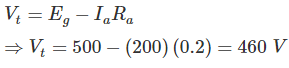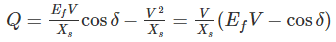# Test: Electrical Machines- 3

## 20 Questions MCQ Test Mock Test Series for SSC JE Electrical Engineering | Test: Electrical Machines- 3

Description
Attempt Test: Electrical Machines- 3 | 20 questions in 12 minutes | Mock test for SSC preparation | Free important questions MCQ to study Mock Test Series for SSC JE Electrical Engineering for SSC Exam | Download free PDF with solutions
QUESTION: 1

### In a generator a dynamically induced emf is produced according to:

Solution:

An electrical generator is a machine which converts mechanic energy into electrical energy. It is based on the principle of production of dynamically induced emf

According to Faraday's Laws of Electromagnetic Induction, whenever a conductor cuts magnetic flux, dynamically induced emf is produced in it. This emf causes a current to flow in the conductor if circuit is closed.

QUESTION: 2

### The EMF induced in the armature of a shunt generator is 500 V. The armature resistance is 0.2 Ω if the armature current is 200 A, what is the terminal voltage?

Solution:

Given that, Eg = 500 V, Ra = 0.2 Ω, Ia = 200 AQUESTION: 3

### In case of four pole, lap wound machine if the air gap under each pole is the same, then what will be the result?

Solution:

In case of lap wound, number of parallel paths (A) = number of poles (P)

The current in the each path will be same.

The terminal voltage will be higher in wave wound machine

In lap wound machine, the hysteresis losses will be reduced whereas eddy current losses will be reduced in wave wound machine

QUESTION: 4

In d.c. generator armature reaction is produced by:

Solution:

There are two kinds of magnetic fluxes acting in DC generator.

1. The flux produced because of the stator poles called main flux

2.  The flux produced because of the current flowing in the armature called armature flux

This armature flux weakens and distort the main flux. Thus the overall effective flux in DC generator gets decrease. This mutual action of armature flux on the main field flux is known as armature reaction.

QUESTION: 5

Which of the following is not necessarily the advantage of D.C motor over A.C Motor?

Solution:

DC motors have the advantage of

1. Higher starting torque

2. Quick starting and stopping, reversing

3. Variable speeds with voltage input and they are easier to control than AC

QUESTION: 6

Solution:

In a differentially compound DC motor at higher values of load, the flux reduces so sharply at small increase in load. It is advisable that motor should not be used beyond some load value, as it may damage itself by self-loading.

QUESTION: 7

The no load primary input is approximately equal to the:

Solution:

When transformer is operated at no load, its secondary winding is open circuited or no load is connected to the secondary winding and hence no current flows through it.

When we apply a primary input voltage, the current produced in the primary winding will supply the core losses (ohmic loss are almost negligible) and would magnetize the core producing mutual flux. This current would be very small in magnitude as it induces a back emf in the primary winding.

QUESTION: 8

Booster transformer are used to:

Solution:

Booster transformer is used towards the end of a power line to raise the voltage to the desired value. It is used for controlling the voltage of a feeder at a point far away from the main transformer.

The secondary of the booster transformer is connected in series with the line, and its primary is supplied from the secondary of the regulating transformer.

Booster transformer is used in railways for eliminating the flow of stray current. The stray current disturbs the communication system and also damage the electronic devices of the trains passing through them.

QUESTION: 9

How the leakage flux is minimized in an actual transformer?

Solution:

We can minimize the leakage flux in a transformer by following methods

1) By reducing the magnetizing current to the minimum

2) By reducing the reluctance of the iron core to the minimum

3) By reducing the number of primary and secondary turn to the minimum

4) By sectionalizing and interleaving the primary and secondary windings

QUESTION: 10

Which test on transformer provides information about regulation efficiency and heating under load conditions?

Solution:

Back to back test on transformer is a method for determining transformer efficiency, voltage regulation and heating under loaded conditions. In this method, two identical transformers are connected back to back such that their primaries are in parallel across the same voltage source and the secondaries in series so that one transformer is loaded on the other.

Short circuit and open circuit tests on transformer can give us parameters of equivalent circuit of transformer but they cannot help us in finding the heating information.

QUESTION: 11

Synchronous generator can _________ reactive power:

Solution:

The reactive power of a synchronous generator is given by,1) When EfV = cosδ then generator works under unity power factor condition

2) When EfV > cosδ then reactive power will be positive, i.e. generator supplies reactive power

3) When EfV < cosδ then reactive power will be negative, i.e. generator absorbs reactive power

QUESTION: 12

To reduce the peripheral speed of an alternator, diameter of the rotor is:

Solution:

Peripheral speed refers to the speed on the periphery or the circumference of the rotor. The peripheral speed is given by the product of the circumference and the speed.

Ps = π × D × RPM

The peripheral speed is dependent on the speed as well as the diameter of the rotor.

Hence to reduce the peripheral speed of an alternator, we need to reduce the diameter of the rotor and increase the axial length.

QUESTION: 13

The Statement “Generators are Coherent”, implies that:

Solution:

‘’Generators are Coherent’’ implies that all the generators connected in parallel to a common bus are oscillating at same frequency.

When two alternators are connected in parallel, their frequency, terminal voltage and phase angle should be equal to busbar’s frequency, terminal voltage and phase angle.

QUESTION: 14

A 3-phase induction motor when started picks up speed but runs stably at about half the normal speed. This is because of:

Solution:

A 3-phase induction motor when started picks up speed but runs stably at about half the normal speed. This happens because of the non-sinusoidal nature of the supply voltage.

QUESTION: 15

Slip rings and brushes are found in:

Solution:

In squirrel cage type rotors, the rotor bar is permanently shorted at the end of the ring. Hence slip rings and brushes are not present in these type of rotors

Slip rings and brushes are present in wound type rotor

QUESTION: 16

For an induction motor the torque is proportional to the product of:

Solution:

The torque of an induction motor is,

T∝ϕI2cosϕ2

Where, ϕ is the flux

Iis rotor current at standstill condition

ϕis the angle between rotor emf and rotor current

QUESTION: 17

What does happen if tripled harmonics given to induction motor?

Solution:

When a sinusoidal input is applied to electronic circuit the output is distorted wave form. This distorted wave is because of nonlinear characteristics of electronic circuit. Those distorted wave is called harmonics. The component having triple times of the fundamental are known as third harmonics. Generally all third harmonics are equal in all phases.

When these harmonics is applied to induction motor, it will fail to start because rotating magnetic field won’t develop.

QUESTION: 18

Which of the following is true for a synchronous condenser?

Solution:

Synchronous condenser is an over-excited synchronous motor operating at no-load with leading power factor.

The efficiency of long power transmission lines may be increased by placing synchronous condensers along the line to compensate lagging currents caused by line inductance.

More real power may be transmitted through a fixed size line if the power factor is brought closer to unity by synchronous condensers absorbing reactive power.

The ability of synchronous condensers to absorb or produce reactive power on a transient basis stabilizes the power grid against short circuits and other transient fault conditions

QUESTION: 19

Which of the following is an unexcited single phase synchronous motor?

Solution:

Unexcited single phase synchronous motor runs at constant speed equal to synchronous speed of revolving flux. They do not need a dc excitation for their rotors.

Reluctance motor and Hysteresis motors are unexcited single phase synchronous motors.

QUESTION: 20

The common type of servo motor is different from normal induction motor as it has:

Solution:

The differences between servo motor and induction motor are:

1) Servo motor is closed loop system where as induction motor is an open loop system

2) An induction motor has high inertia and servo motor has a very low inertia. Hence servo motors are used in applications where instant and accurate positioning of load is required

3) Servo motor has high rotor resistance and low power rating compared to induction motorUse Code STAYHOME200 and get INR 200 additional OFF Use Coupon Code